# TSA AR Spectrum VI

LabVIEW 2014 Advanced Signal Processing Toolkit Help

Edition Date: June 2014

Part Number: 372656C-01

»View Product InfoDownload Help (Windows Only)

Owning Palette: Correlation and Spectral Analysis VIs

Computes the single-sided power spectral density (PSD) of a univariate time series based on autoregressive (AR) modeling. The PSD computed with this method is exempt from window effects and has a better frequency resolution than the results from using the periodogram method.

Wire data to the Xt input to determine the polymorphic instance to use or manually select the instance.

Use the pull-down menu to select an instance of this VI.

 Select an instance TSA AR Spectrum (Waveform)TSA AR Spectrum (Array)

## TSA AR Spectrum (Waveform)frequency bins specifies the number of frequency bins for which this VI computes the single-sided power spectral density PSD. The length of the single-sided PSD is (frequency bins/2+1). The default is 1024.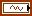Xt specifies the univariate time series.AR method specifies the method this VI uses to estimate the autoregressive (AR) model.

 0 Forward-Backward (default)—Computes the AR coefficients by minimizing the least-square errors of the forward and backward predictions. 1 Least-Squares—Computes the AR coefficients by minimizing the least-square errors of the forward predictions. 2 Yule-Walker—Computes the AR coefficients by solving the Yule-Walker functions based on the forward predictions. 3 Burg-Lattice—Computes the AR coefficients using the Levinson-Durbin recursion based on the forward and backward predictions. The Levinson-Durbin recursion uses the arithmetic average. 4 Geometric-Lattice—Computes the AR coefficients using the Levinson-Durbin recursion based on the forward and backward predictions. The Levinson-Durbin recursion uses the geometric average.AR order specifies the order of the autoregressive (AR) model. The value of AR order must be greater than 0. The default is 4.error in describes error conditions that occur before this node runs. This input provides standard error in functionality.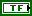dB on? specifies whether this VI returns the PSD in decibels or in a linear scale. If dB on? is TRUE, this VI returns the PSD in decibels. If dB on? is FALSE, this VI returns the PSD in a linear scale. The default is TRUE.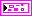PSD returns information about the single-sided power spectral density (PSD).f0 returns the lower boundary, in hertz, of the frequency range.df returns the frequency increment, in hertz.S(f) returns the magnitude of the PSD at each frequency. The value of dB on? determines the unit of measurement for this parameter.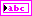unit returns the engineering unit of the PSD. You can specify an engineering unit for a time series by using the TSA Scale to EU VI.error out contains error information. This output provides standard error out functionality.

## TSA AR Spectrum (Array)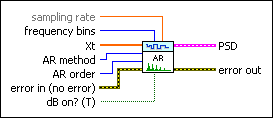sampling rate specifies the sampling rate, in hertz, of the univariate time series Xt. The default is 1.frequency bins specifies the number of frequency bins for which this VI computes the single-sided power spectral density PSD. The length of the single-sided PSD is (frequency bins/2+1). The default is 1024.Xt specifies the univariate time series.AR method specifies the method this VI uses to estimate the autoregressive (AR) model.

 0 Forward-Backward (default)—Computes the AR coefficients by minimizing the least-square errors of the forward and backward predictions. 1 Least-Squares—Computes the AR coefficients by minimizing the least-square errors of the forward predictions. 2 Yule-Walker—Computes the AR coefficients by solving the Yule-Walker functions based on the forward predictions. 3 Burg-Lattice—Computes the AR coefficients using the Levinson-Durbin recursion based on the forward and backward predictions. The Levinson-Durbin recursion uses the arithmetic average. 4 Geometric-Lattice—Computes the AR coefficients using the Levinson-Durbin recursion based on the forward and backward predictions. The Levinson-Durbin recursion uses the geometric average.AR order specifies the order of the autoregressive (AR) model. The value of AR order must be greater than 0. The default is 4.error in describes error conditions that occur before this node runs. This input provides standard error in functionality.dB on? specifies whether this VI returns the PSD in decibels or in a linear scale. If dB on? is TRUE, this VI returns the PSD in decibels. If dB on? is FALSE, this VI returns the PSD in a linear scale. The default is TRUE.PSD returns information about the single-sided power spectral density (PSD).f0 returns the lower boundary, in hertz, of the frequency range.df returns the frequency increment, in hertz.S(f) returns the magnitude of the PSD at each frequency. The value of dB on? determines the unit of measurement for this parameter.error out contains error information. This output provides standard error out functionality.

## TSA AR Spectrum Details

This VI computes the single-sided PSD of a univariate time series based on AR modeling according to the following equation: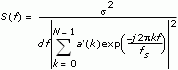where S(f) is the PSD of the time series. df is the frequency interval, which is computed as fs/N. N is the number of frequency bins, fs is the sampling rate, and s2 is the noise variance of the estimated AR model of the time series. a is an array that contains the coefficients of the AR model. a=[1, a1, a2, … ,an], where n is AR order. Before computing the PSD, this VI wraps a to N-point series a'.

The minimum length requirement for the input time series needs to be at least two times the AR order.

## Example

Refer to the Power Spectral Density Estimation VI in the labview\examples\Time Series Analysis\TSAGettingStarted directory for an example of using the TSA AR Spectrum VI.